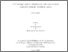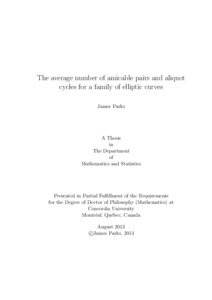Title:

The average number of amicable pairs and aliquot cycles for a family of elliptic curves

Parks, James (2013) The average number of amicable pairs and aliquot cycles for a family of elliptic curves. PhD thesis, Concordia University.Preview
Text (application/pdf)
Parks_PhD_F2013.pdf - Accepted Version
541kB

Abstract

Let E be an elliptic curve over Q. Silverman and Stange defined the set (p_1,...,p_L) of distinct primes to be an aliquot cycle of length L of E if each p_i is a prime of good reduction for E such that #E_{p_1)(F_{p_1})=p_2,...,#E_{p_{L-1}}(F_{p_{L-1}})=p_L, #E_{p_L}(F_{p_L})=p_1. Let \pi_{E,L}(X) denote the aliquot cycle counting function with p<=X. They conjectured for elliptic curves without complex multiplication that
\pi_{E,L}(X) ~ \sqrt{X}/(\log X)^{L}. Jones refined this conjecture to give an explicit constant,
namely \pi_{E,L}~C_{E,L}\sqrt{X}/(\log X)^L. In this thesis we will show that the conjectured upper bound holds for \pi_{E,L}(X) on average over the family of all elliptic curves with a short length for the average.

Divisions: Concordia University > Faculty of Arts and Science > Mathematics and Statistics Thesis (PhD) Parks, James Concordia University Ph. D. Mathematics 27 August 2013 David, Chantal elliptic curves, aliquot cycles, amicable pairs 977734 JAMES PARKS 13 Jan 2014 15:11 18 Jan 2018 17:45
All items in Spectrum are protected by copyright, with all rights reserved. The use of items is governed by Spectrum's terms of access.

Repository Staff Only: item control pageResearch related to the current document (at the CORE website)
Back to top